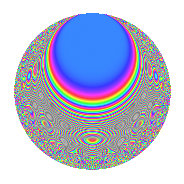# Properties

 Label 54.5.fLevel 54 Weight 5 Character orbit f Rep. character $$\chi_{54}(5,\cdot)$$ Character field $$\Q(\zeta_{18})$$ Dimension 72 Newform subspaces 1 Sturm bound 45 Trace bound 0

# Related objects

## Defining parameters

 Level: $$N$$ $$=$$ $$54 = 2 \cdot 3^{3}$$ Weight: $$k$$ $$=$$ $$5$$ Character orbit: $$[\chi]$$ $$=$$ 54.f (of order $$18$$ and degree $$6$$) Character conductor: $$\operatorname{cond}(\chi)$$ $$=$$ $$27$$ Character field: $$\Q(\zeta_{18})$$ Newform subspaces: $$1$$ Sturm bound: $$45$$ Trace bound: $$0$$

## Dimensions

The following table gives the dimensions of various subspaces of $$M_{5}(54, [\chi])$$.

Total New Old
Modular forms 228 72 156
Cusp forms 204 72 132
Eisenstein series 24 0 24

## Trace form

 $$72q - 18q^{5} - 96q^{6} + 204q^{9} + O(q^{10})$$ $$72q - 18q^{5} - 96q^{6} + 204q^{9} + 720q^{11} + 96q^{12} - 288q^{14} - 1422q^{15} - 1344q^{18} + 288q^{20} + 4308q^{21} - 1008q^{22} + 4716q^{23} - 882q^{25} - 2808q^{27} - 6084q^{29} - 6336q^{30} + 3330q^{31} - 1026q^{33} + 288q^{34} + 5346q^{35} + 2976q^{36} + 576q^{38} - 4974q^{39} - 13356q^{41} - 3840q^{42} + 1260q^{43} + 9558q^{45} + 16578q^{47} + 1920q^{48} - 5904q^{49} + 15552q^{50} + 20898q^{51} - 4896q^{54} - 2304q^{56} - 34218q^{57} - 40104q^{59} - 4176q^{60} + 8352q^{61} - 4110q^{63} + 18432q^{64} - 19674q^{65} - 10368q^{66} - 24192q^{67} + 10224q^{68} + 47106q^{69} + 14400q^{70} + 39528q^{71} + 15360q^{72} - 12222q^{73} + 33120q^{74} + 41682q^{75} + 9792q^{76} + 28206q^{77} - 4608q^{78} + 11304q^{79} + 6804q^{81} - 30078q^{83} - 2592q^{84} - 52200q^{85} - 46224q^{86} - 111690q^{87} - 16128q^{88} - 102222q^{89} - 66240q^{90} + 12078q^{91} - 27504q^{92} - 11958q^{93} + 4032q^{94} + 46728q^{95} + 6144q^{96} + 49680q^{97} + 82944q^{98} + 179622q^{99} + O(q^{100})$$

## Decomposition of $$S_{5}^{\mathrm{new}}(54, [\chi])$$ into newform subspaces

Label Dim. $$A$$ Field CM Traces $q$-expansion
$$a_2$$ $$a_3$$ $$a_5$$ $$a_7$$
54.5.f.a $$72$$ $$5.582$$ None $$0$$ $$0$$ $$-18$$ $$0$$

## Decomposition of $$S_{5}^{\mathrm{old}}(54, [\chi])$$ into lower level spaces

$$S_{5}^{\mathrm{old}}(54, [\chi]) \cong$$ $$S_{5}^{\mathrm{new}}(27, [\chi])$$$$^{\oplus 2}$$

## Hecke characteristic polynomials

There are no characteristic polynomials of Hecke operators in the database